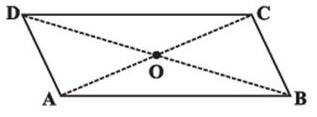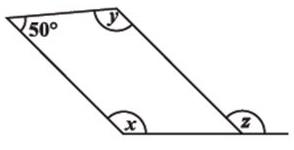Courses

## 10 Questions MCQ Test Mathematics (Maths) Class 8 | Test: Understanding Quadrilaterals- 1

Description
This mock test of Test: Understanding Quadrilaterals- 1 for Class 8 helps you for every Class 8 entrance exam. This contains 10 Multiple Choice Questions for Class 8 Test: Understanding Quadrilaterals- 1 (mcq) to study with solutions a complete question bank. The solved questions answers in this Test: Understanding Quadrilaterals- 1 quiz give you a good mix of easy questions and tough questions. Class 8 students definitely take this Test: Understanding Quadrilaterals- 1 exercise for a better result in the exam. You can find other Test: Understanding Quadrilaterals- 1 extra questions, long questions & short questions for Class 8 on EduRev as well by searching above.
QUESTION: 1

### Which of the following quadrilaterals has two pairs of adjacent sides equal and diagonals intersecting at right angles?

Solution:

quadrilateral is a rhombus if: it is a parallelogram, and a pair of adjacent sides are equal, its diagonals bisect each other at right angles, its diagonals bisect each vertex angle.

QUESTION: 2

### If the sides of a triangle are produced in order, Prove that the sum of the exterior angles so formed is 360°.

Solution:

Suppose you have a ∆ABC
All the exterior angles will be equal to
(180-A)°
(180-B)°
(180-C)°
respectively (Angles on the same line) so if you sum it up it will be equal to 540 - (A+ B + C )
And by angle sum property A+B+C = 180°
Therefore Sum = 540 - 180 = 360°

QUESTION: 3

### Given a parallelogram ABCD. AD = ....Solution: BC ( because of opposite sides of the parallelogram are equal = )
QUESTION: 4

The sum of the measures of the three angles of a triangle is ______.

Solution:

Angle sum property of a triangle is that sum of 3 angles is 180º

QUESTION: 5

The ________ of a rhombus are perpendicular bisectors of one another.

Solution:
QUESTION: 6

Find the value of the unknown x in this parallelogram.Solution:
QUESTION: 7

The opposite sides of a parallelogram are of ________ length.

Solution:

It’s a property of parallelogram that opposite sides of a parallelogram are equal and parallel.

QUESTION: 8

Which of the parallelograms has all sides equal and diagonals bisect each other at right angle?

Solution:

The diagonals of a parallelogram only bisect each other at right angles if the parallelogram is a rhombus.

QUESTION: 9

Which of the following quadrilaterals has two pairs of adjacent sides equal and diagonals intersecting at right angles?

Solution:
Sum of the exterior angles of regular polygon = 360degree

But each exterior angle = 45degree

number of sides of regular polygon = 360degree/ 45degree = 8.

QUESTION: 10

State the name of a regular polygon of 5 sides.

Solution:

Pentagon have 5 sides .